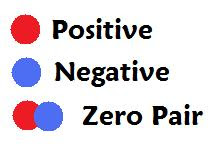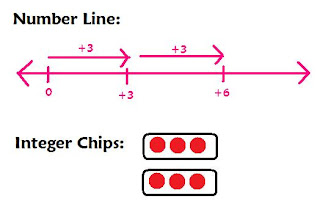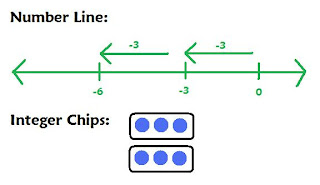## Friday, March 11, 2011

### Melanie's Great Big Book of Integers

Chapter 1: Grade 7 Integer Review

In class, we discussed the different ways you can solve integer questions. The two ways are:
• using a number line
• using integer chips* Remember the rule, " When subtracting something that isn't there, use a zero pair."

Here are some
examples of integer questions (adding and subtracting) :

1. -3 - (-7) = +42. -3 -7 = -103. 3 - 7 = +104. 3 + 7 = +105. -3 +7 = +4Chapter 2: Multiplying Integers

* A rule that will help you with multiplying integers is "The Sign Rule."
This rule states that:
• Whenever you have an even number of negative factors, the product is positive.
• And when you have an odd number of negative factors, the product is negative
* Remember that you can also use number lines when solving multiplication integer questions, you just can't use them when the first number of the equation is a negative such as -6.

Here are some examples of multiplication integer questions:

1. (+2) x (+3) = +62. (+2) x (-3) = -63. (-2) x (+3) = -64. (-2) x (-3) = +6Chapter 3: Dividing Integers

* Partitive Division is spreading a the quotient into parts or groups.
Here are examples of partitive division using a number line:* Quotative Division is sharing the total with groups.

Example:* The sign rule can really help you when solving integer questions.

* Reminder: The sign rule states that when you have an odd number of negative signs, the answer is negative but if there are an even number of negative signs, the answer is positive.
For example: 1) 6÷2= +3
I knew this because the question had 0 negative signs which makes the answer a positive number.

2) -6 ÷ (-2)= +3
I knew that the answer is positive because there are 2 negative signs.

3) (-6)÷2= -3
There is an only 1 negative sign which means that the answer has to be negative.

4) 6÷(-2)= -3
The question has 1 negative sign which let me know that the answer is a negative.

Chapter 4: Order of Operations with Integers

Example:
(+5) x (-3) + (-6) ÷ (+3)=* Remember that when solving questions such as the one above, you should use BEDMAS.

B= brackets
E= exponents
D= division
M=
multiplication
A=
S=
subtraction

*Here are the steps for solving questions such as the example above:

1) First you add square brackets around anything that needs to be multiplied or divided.

2) Then you multiply or divide whatever is in the square brackets.

3) You then do the same steps to whatever remaining numbers with the operation of multiplication or division.

4) Once completed that step, you then move on to addition and subtraction and just simply do the same.

* Remember to always move left to right when using BEDMAS.